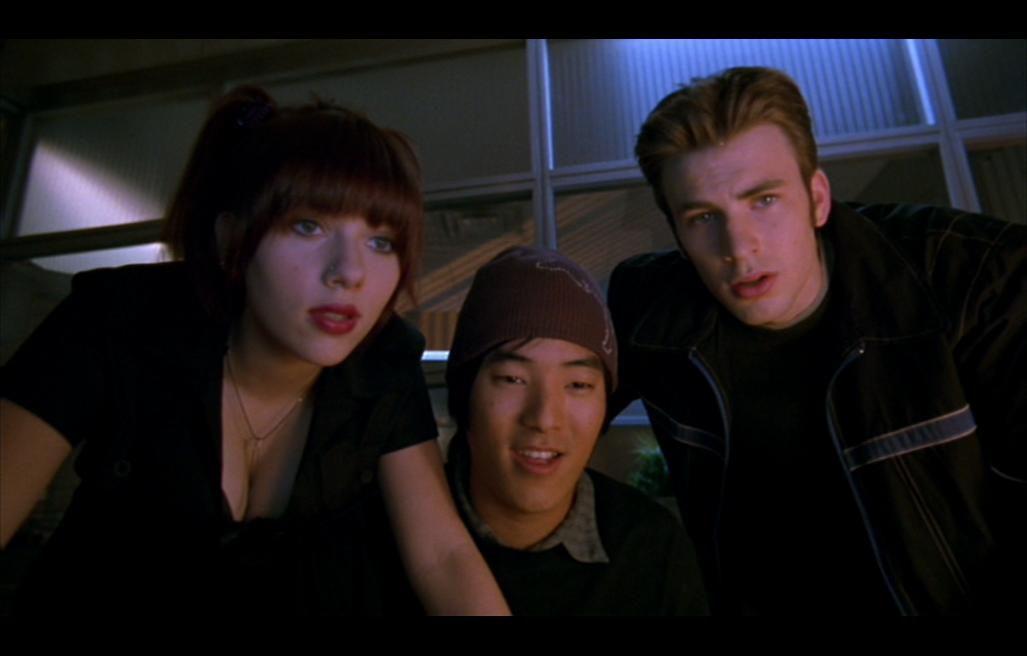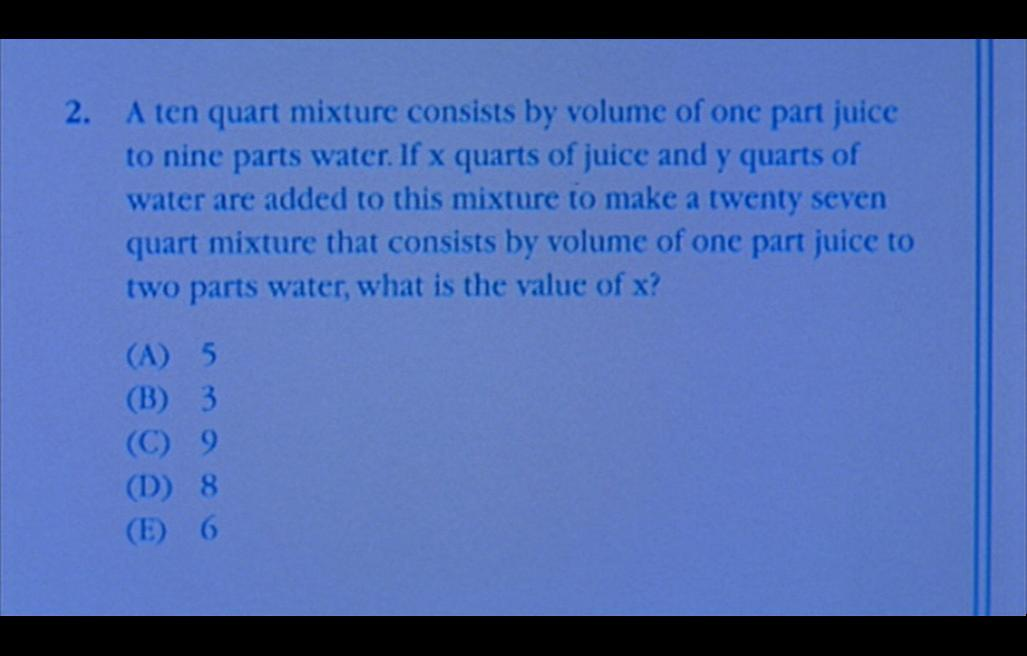Fall 2004

# Mathematics Math21b Fall 2004

## Linear Algebra and Differential Equations

Office: SciCtr 434
Email: knill@math.harvard.edu# A SAT problem in "perfect score"

In the movie "Perfect Score" (Paramount 2004), the following SAT problem appears:

 "A ten quart mixture consists by volume of one part juice and 9 parts water. If x quarts of juice and y quarts of water are added to this mixture to make a 27 quart mixture that consists by volume of one part juice to two parts water, what is the value of x? "

The problem already gave the variables x=quarts of juice and y =quarts of water which are added. After adding we have 1+x juice and 9+y water. One part of the problem tells that the sum 10+x+y is 27. The second part of the problem is that 1/3 of it is water which means (10+x+y)/(1+x) = 3. Formally this is a system of linear equations
```     10+x+y = 27
3(1+x) = 10+x+y
```
with solution x=8 and y=9. Tis can of course also be solved quickly from knowing that the total amount is 27 and a third of it is 1+x. So that 1+x=9 or x=8. The variable y is not needed in this short cut at all.Back to the main page